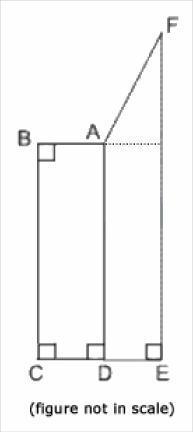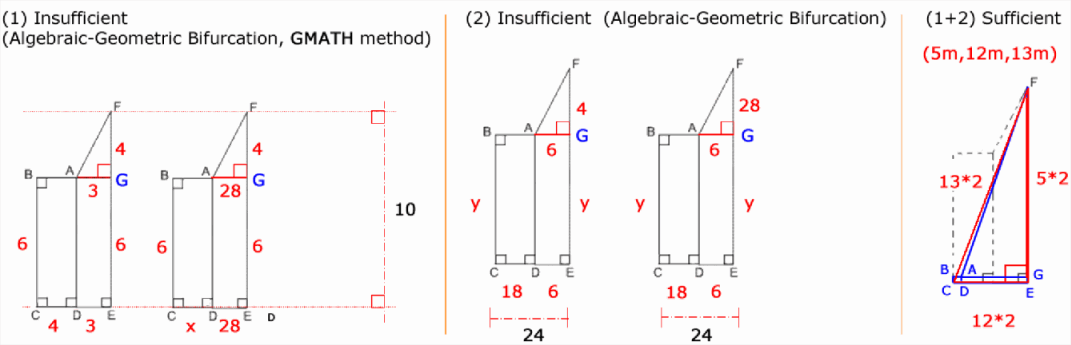GMAT Question of the Day - Daily to your Mailbox; hard ones only

 It is currently 19 May 2019, 06:22### GMAT Club Daily Prep

#### Thank you for using the timer - this advanced tool can estimate your performance and suggest more practice questions. We have subscribed you to Daily Prep Questions via email.

Customized
for You

we will pick new questions that match your level based on your Timer History

Track
Your Progress

every week, we’ll send you an estimated GMAT score based on your performance

Practice
Pays

we will pick new questions that match your level based on your Timer History

#### Not interested in getting valuable practice questions and articles delivered to your email? No problem, unsubscribe here.### Request Expert Reply# If quadrilaterals ABCD and ADEF have the same areas, is AF>28?

 new topic post reply Question banks Downloads My Bookmarks Reviews Important topics
Author Message
TAGS:

### Hide Tags

GMATH TeacherP
Status: GMATH founder
Joined: 12 Oct 2010
Posts: 934
If quadrilaterals ABCD and ADEF have the same areas, is AF>28?  [#permalink]

### Show Tags

1
100:00

Difficulty:65% (hard)

Question Stats:51% (02:28) correct49% (01:46) wrongbased on 37 sessions

### HideShow timer Statistics

GMATH practice exercise (Quant Class 15)If quadrilaterals ABCD and ADEF have the same areas, is AF > 28 ?

(1) EF = 10
(2) CE = 24

Edited:
01. The contest related to this post is finished!
02. We have presented the official solution.
03. We have added the dotted line, to avoid the interpretation that F could be "below" line AB.

_________________
Fabio Skilnik :: GMATH method creator (Math for the GMAT)
Our high-level "quant" preparation starts here: https://gmath.net

Originally posted by fskilnik on 15 Mar 2019, 10:55.
Last edited by fskilnik on 17 Mar 2019, 07:00, edited 3 times in total.
ManagerG
Joined: 01 Feb 2017
Posts: 222
Re: If quadrilaterals ABCD and ADEF have the same areas, is AF>28?  [#permalink]

### Show Tags

1
IMO, Ans C

Considering both statements together and a rectangle with base CE and height FE, resultant area 240.

So, area of each quad is less than / equal to 240/2= 120 max.

In trapezium / rectangle ADEF, FE is 10, so average of FE+AD is less than or equal to 10. So, max possible value of DE is 12.

In triangle DEF with base 12 and height 10, Hyp FD is root 244, approx 15.something.
As AF is smaller than FD, AF cannot be greater than 28.
InternB
Joined: 06 Feb 2019
Posts: 6
Re: If quadrilaterals ABCD and ADEF have the same areas, is AF>28?  [#permalink]

### Show Tags

(1) EF = 10

We don't know the length of DE. You can stretch DE very far apart (could be to a ridiculous number, say to 1 million) then clearly AF, which forms the hypotenuse of the right triangle with base the length of DE & height < 10 would be greater than 28. However, if DE is very short, such as length of 1, then AF is the hypotenuse of a right triangle with other sides 1 & a side less than 10, so AF is less than 28.

Insufficient

(2) CE = 24

A similar thought exercise can be performed here, where if EF is a ridiculously long length, then you can construct AF to be >28, but if EF is sufficiently short enough, then AF < 28.

Insufficient

(Both 1 & 2)

Knowing EF is 10 and CE is 24, we can try to maximize the length of AF to see if it exceeds 28. If it doesn't, then we know the answer is sufficient.

We can set BA and AD to be very small, almost 0, such that AF forms the hypotenuse of a right triangle with sides 10 and 24, which a quick calculation shows that at most AF can be 26. So AF is definitely less than 28.

Answer is C
GMATH TeacherP
Status: GMATH founder
Joined: 12 Oct 2010
Posts: 934
If quadrilaterals ABCD and ADEF have the same areas, is AF>28?  [#permalink]

### Show Tags

The contest is finished from this moment on!

We thank Shobhit7 and siyeezy for your beautiful contributions!

We will start commenting on both solutions (separately) based on our judge´s considerations:

Shobhit7 wrote:
IMO, Ans C

Considering both statements together and a rectangle with base CE and height FE, resultant area 240.

So, area of each quad is less than / equal to 240/2= 120 max.

In trapezium / rectangle ADEF, FE is 10, so average of FE+AD is less than or equal to 10. So, max possible value of DE is 12.

In triangle DEF with base 12 and height 10, Hyp FD is root 244, approx 15.something.
As AF is smaller than FD, AF cannot be greater than 28.

Shobhit:

01. You did not prove each statement alone is insufficient ("it´s obvious" is not a good argument, at least in a prize-winning contest)...
02. Area of each quadrilateral must be LESS than 240/2, because from the figure given points B, A, and F should be considered NOT collinear in Data Sufficient situations like this one!
03. Nice arguments using the "middle-base" of the trapezoid (although, again, DE must be less than 12, because 10=FE>AD) and hypotenuse FD, offering an upper limit to AF <<< 28 !!

siyeezy wrote:
(1) EF = 10

We don't know the length of DE. You can stretch DE very far apart (could be to a ridiculous number, say to 1 million) then clearly AF, which forms the hypotenuse of the right triangle with base the length of DE & height < 10 would be greater than 28. However, if DE is very short, such as length of 1, then AF is the hypotenuse of a right triangle with other sides 1 & a side less than 10, so AF is less than 28.

Insufficient

(2) CE = 24

A similar thought exercise can be performed here, where if EF is a ridiculously long length, then you can construct AF to be >28, but if EF is sufficiently short enough, then AF < 28.

Insufficient

(Both 1 & 2)

Knowing EF is 10 and CE is 24, we can try to maximize the length of AF to see if it exceeds 28. If it doesn't, then we know the answer is sufficient.

We can set BA and AD to be very small, almost 0, such that AF forms the hypotenuse of a right triangle with sides 10 and 24, which a quick calculation shows that at most AF can be 26. So AF is definitely less than 28.

Answer is C

siyeezy:

01. Very nice viable "geometric bifurcations" (using the term we have created in our method) to guarantee the insufficiency of each statement alone.
02. Saw the important right triangle 10,24,26 (we consider "5m,12m,13m" a GMAT-shortcut!) but did not mention *explicitly* that the "equal areas restriction" would be temporarily ignored for that. The fact that the upper limit (26) is already less than 28 (our FOCUS) makes this approach excellent because (according to our method) the FOCUS is the most important guide to our decisions!

All that put, the judge (and I) believe both contributions were REALLY great, therefore we will consider... a DRAW! Both of you will receive the prize we had in mind: our "Lightning" course!

I hope you will be able to strengthen even more your powerful skills through our method!

Congratulations to both of you and thanks for joining!!
Fabio.

P.S.: I will wait a private message from each one of you, to give the step-by-step instructions for collecting the prize! Cheers!
_________________
Fabio Skilnik :: GMATH method creator (Math for the GMAT)
Our high-level "quant" preparation starts here: https://gmath.net
GMATH TeacherP
Status: GMATH founder
Joined: 12 Oct 2010
Posts: 934
If quadrilaterals ABCD and ADEF have the same areas, is AF>28?  [#permalink]

### Show Tags

1
fskilnik wrote:
GMATH practice exercise (Quant Class 15)If quadrilaterals ABCD and ADEF have the same areas, is AF > 28 ?

(1) EF = 10
(2) CE = 24$${S_{{\mathop{\rm ABCD}\nolimits} }} = {S_{{\rm{ADEF}}}} = {S_{{\rm{ADEG}}}} + {S_{{\rm{AGF}}}}\,\,\,\,\,\left( * \right)$$

$$AF\,\,\mathop > \limits^? \,\,28$$

$$\left( 1 \right)\,\,\left\{ \matrix{ \,{\rm{image}}\,\,{\rm{left}}:\,\,\left( * \right)\,\,4 \cdot 6 = 3 \cdot 6 + {{3 \cdot 4} \over 2}\,\,\,\,\, \Rightarrow \,\,\,\,{\rm{viable}}\,\,\,\, \Rightarrow \,\,\,\,\left\langle {{\rm{NO}}} \right\rangle \hfill \cr \,{\rm{image}}\,\,{\rm{right}}:\,\,\left( * \right)\,\,x \cdot 6 = 28 \cdot 6 + {{28 \cdot 4} \over 2}\,\,\,\,\, \Rightarrow \,\,\,\,{\rm{viable}}\,\,\,\left( {x = {{28 \cdot 8} \over 6} > 0} \right)\,\,\,\, \Rightarrow \,\,\,\,\left\langle {{\rm{YES}}} \right\rangle \hfill \cr} \right.$$

$$\left( 2 \right)\,\,\left\{ \matrix{ \,{\rm{image}}\,\,{\rm{left}}:\,\,\left( * \right)\,\,18 \cdot y = 6 \cdot y + {{6 \cdot 4} \over 2}\,\,\,\,\, \Rightarrow \,\,\,\,{\rm{viable}}\,\,\,\,\left( {y = 1 > 0} \right) \Rightarrow \,\,\,\,\left\langle {{\rm{NO}}} \right\rangle \hfill \cr \,{\rm{image}}\,\,{\rm{right}}:\,\,\left( * \right)\,\,18 \cdot y = 6 \cdot y + {{6 \cdot 28} \over 2}\,\,\,\,\, \Rightarrow \,\,\,\,{\rm{viable}}\,\,\,\left( {y = 7 > 0} \right)\,\,\,\, \Rightarrow \,\,\,\,\left\langle {{\rm{YES}}} \right\rangle \hfill \cr} \right.$$

$$\left( {1 + 2} \right)\,\,{\rm{Extremal}}\,\,{\rm{Scenario}}\,\,\,\left( {\left( * \right)\,\,{\rm{ignored}}} \right)\,\,:\,\,A{F_{\,{\rm{ExtScen}}}}\,\,\mathop < \limits^{{\rm{near}}} \,\,13 \cdot 2 = 26$$

$$\Rightarrow \,\,AF < \,\,A{F_{\,{\rm{ExtScen}}}} < 28\,\,\,\, \Rightarrow \,\,\,\,{\rm{SUFF}}.$$

The correct answer is (C).

We follow the notations and rationale taught in the GMATH method.

Regards,
Fabio.
_________________
Fabio Skilnik :: GMATH method creator (Math for the GMAT)
Our high-level "quant" preparation starts here: https://gmath.netIf quadrilaterals ABCD and ADEF have the same areas, is AF>28?   [#permalink] 17 Mar 2019, 07:18
Display posts from previous: Sort by

# If quadrilaterals ABCD and ADEF have the same areas, is AF>28?

 new topic post reply Question banks Downloads My Bookmarks Reviews Important topicsPowered by phpBB © phpBB Group | Emoji artwork provided by EmojiOne Kindly note that the GMAT® test is a registered trademark of the Graduate Management Admission Council®, and this site has neither been reviewed nor endorsed by GMAC®.

#### MBA Resources